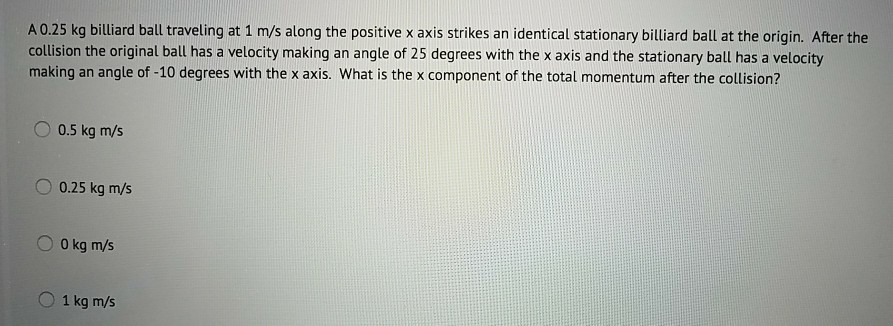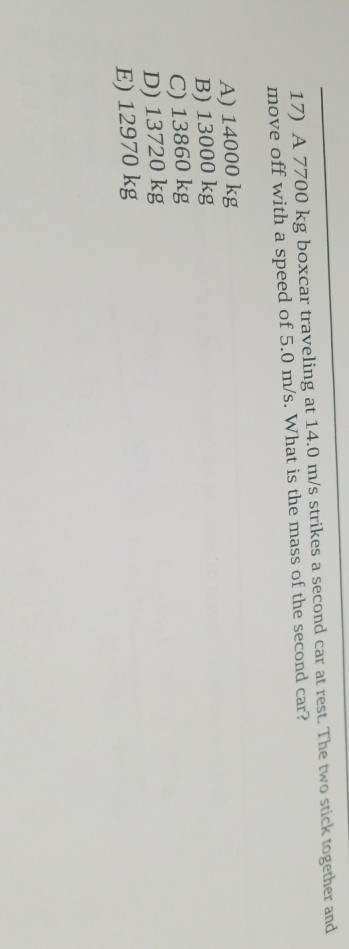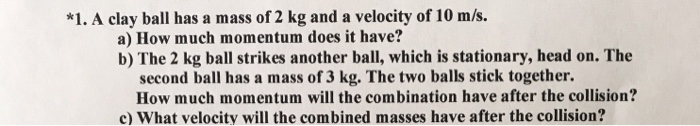# A 2,000 kg car traveling north at a velocity of 45m/s strikes 2,200 Kg car traveling...

A 2,000 kg car traveling north at a velocity of 45m/s strikes 2,200 Kg car traveling west at 50 m/s. The two vehicles strike each other and stick together. As a single combined mass they slide across the pavement which has a coefficient of kinetic friction 0.07. What is the displacement from the point of impact to the final resting place of the cars? (How long does it take for them to come to rest?)

A 200 Kg ball is moving west at 20m/s. It elastically strikes a stationary 300Kg ball. Ignore any effect of friction, what is the final velocity of the balls.

1) In X direction

=> - 2200 * 50   = (2000 + 2200) * vx

=> vx = - 26.19 m/sec

In Y direction

=> 2000 * 45 = (2000 + 2200) * vy

=> vy = 21.428 m/sec

=> Resultant velocity = 33.83 m/sec

=>   displacement from the point of impact to the final = (33.83)2/(2 * 0.07 * 9.8)

= 834.16 m

=>    long does it take for them to come to rest   =   (33.83)/(0.07 * 9.8)

= 49.31 sec

2)   Here,   - 200 * 20 = 200v1 + 300v2

Also,    -20 = v2 - v1

=> final velocity of 200 kg ball = 4 m/sec     (towards east)

=>   final velocity of 300 kg ball = - 16 m/sec    (towards west)

#### Earn Coin

Coins can be redeemed for fabulous gifts.

Similar Homework Help Questions
• ### A small compact car with a mass of 1000 kg is traveling north towards an intersection...

A small compact car with a mass of 1000 kg is traveling north towards an intersection at a speed of 15 m/s. A truck with a mass of 2000 kg is traveling east at 10 m/s on the cross street approaching the same intersection. The two vehicles collide and stick together. a. What is the magnitude and direction of the velocity of both vehicles after the collision? b. How much energy was lost during the collision?

• ### A 1000·kg car traveling with a velocity of 13·m/s north collides head-on with a 2000·kg truck traveling with a velocity of 17·m/s south

A 1000·kg car traveling with a velocity of 13·m/s north collides head-on with a 2000·kg truck traveling with a velocity of 17·m/s south. Immediately after the collision, the velocity of the car is 11·m/s south. Taking north as the positive direction, what is the velocity of the truck immediately after the collision?

• ### A 1200·kg car traveling with a velocity of 12·m/s north collides head-on with a 2400·kg truck...

A 1200·kg car traveling with a velocity of 12·m/s north collides head-on with a 2400·kg truck traveling with a velocity of 8·m/s south. Immediately after the collision, the velocity of the car is 4·m/s south. Take north as the positive direction (a) What is the velocity of the truck immediately after the collision? (b) What impluse is delivered to the car by the truck? (c) What impluse is delivered to the truck by the car?

• ### A 900 kg car traveling east at 15.0 m/s suddenly collides with a 750 kg car...

A 900 kg car traveling east at 15.0 m/s suddenly collides with a 750 kg car traveling north at 20.0 m/s. The cars stick together after the collision. What is the velocity of the wreckage move just after the collision?

• ### A 900-kg car traveling east at 15.0 m/s collides with a 750-kg car traveling north at...

A 900-kg car traveling east at 15.0 m/s collides with a 750-kg car traveling north at 20.0 m/s. The cars stick together. Assume that any other unbalanced forces are negligible. a. What is the speed of the wreckage just after the collision? b. In what direction does the wreckage move just after the collision? c. How much kinetic energy is lost in this collision.

• ### A 0.25 kg billiard ball traveling at 1 m/s along the positive x axis strikes an...A 0.25 kg billiard ball traveling at 1 m/s along the positive x axis strikes an identical stationary billiard ball at the origin. After the collision the original ball has a velocity making an angle of 25 degrees with the x axis and the stationary ball has a velocity making an angle of -10 degrees with the x axis. What is the x component of the total momentum after the collision? O 0.5 kg m/s 0.25 kg m/s O 0...

• ### 17) A 7700 kg boxcar traveling at 14.0 m/s strikes a second car at rest. The...17) A 7700 kg boxcar traveling at 14.0 m/s strikes a second car at rest. The two stick together and move off with a speed of 5.0 m/s. What is the mass of the second car? A) 14000 kg B) 13000 kg C) 13860 kg D) 13720 kg E) 12970 kg

• ### *1. A clay ball has a mass of 2 kg and a velocity of 10 m/s....*1. A clay ball has a mass of 2 kg and a velocity of 10 m/s. a) How much momentum does it have? b) The 2 kg ball strikes another ball, which is stationary, head on. The second ball has a mass of 3 kg. The two balls stick together. How much momentum will the combination have after the collision? c) What velocity will the combined masses have after the collision?

• ### A 1200 kg car traveling 45.0o south of east at 18.0 m/s collides with a 850...

A 1200 kg car traveling 45.0o south of east at 18.0 m/s collides with a 850 kg car traveling north at 23.0 m/s. The cars stick together. What is the velocity of the wreckage just after the collision?

• ### A 1000-kg car approaches an intersection traveling north at 15.0 m/s. A 1300-kg car approaches the...

A 1000-kg car approaches an intersection traveling north at 15.0 m/s. A 1300-kg car approaches the same intersection traveling in the same direction behind first car at at 28.0 m/s. The two cars collide at the intersection and lock together. Ignoring any external forces that act on the cars during the collision, what is the velocity of the cars immediately after the collision?

Free Homework App